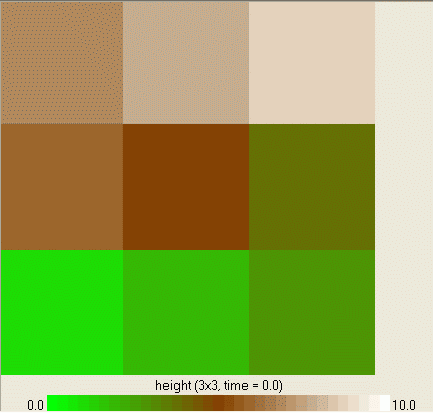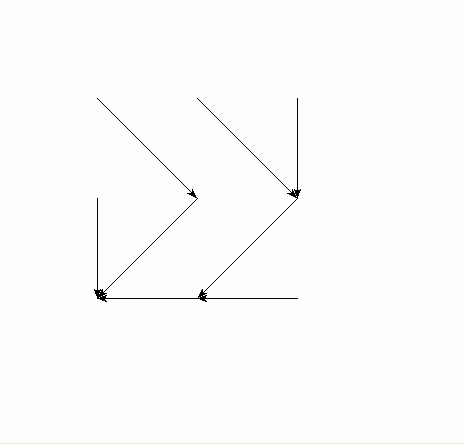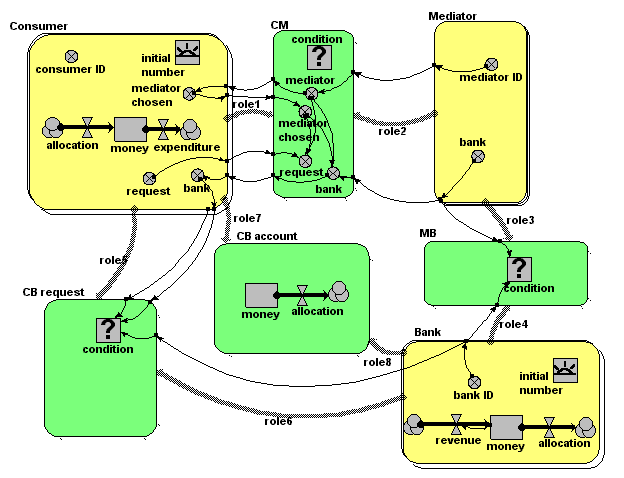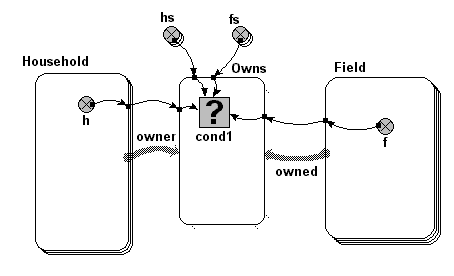# Fixed-membership submodel

## Food-web modelling: two trophic layers

ModelId:
feeding1
SimileVersion:
3.1+

This model simulates the feeding relationships between two trophic layers: plants and herbivores. Each trophic layer is represented in terms of a number of species, and we model the feeding between (potentially) each species at one level and each species on the other level. An association submodel is used to specify which species of herbivore actually feeds on which species of plant.## Embryo morphogenesis

ModelId:
embryo1
SimileVersion:
3.1+

This model implements ideas developed by John Gurdon, Cambridge University, on the role of activin in morphogenesis. The following gives a biological statement about the system on the left, and the corresponding Simile realisation on the right.

Results:

The animation below shows the activin concentration on the left; the SMAD concentrations in the middle, and the resulting cell commitment to cell type on the right.## Drainage pattern

ModelId:
drainage1
SimileVersion:
4.0

A classic spatial problem is calculating the drainage pattern for a given land area. If the land is divided in to cells in a grid with the altitude of the land in each cell being known the law the drainage direction for each cell can be found by finding the neighbour with the lowest altitude.

Equations:

Equations in drainage1

Variable h : Height array
h = [height]
Where:
[height]=cell/height
Outside the cell submodel to provide an array of heights the elements of which are addressable from inside /cell and "/cell/neighbours and self" . (Inside a submodel only values for the current instance is accessible.)

Equations in cell

Variable col
col = index(1)-(row-1)*3

Variable flow direction : Bearing from north to lower neighbour (degrees)
flow direction = if nid_min==1 then 225 elseif nid_min==2 then 180 elseif nid_min==3 then 135 elseif nid_min==4 then 270 elseif nid_min==6 then 90 elseif nid_min==7 then 315 elseif nid_min==8 then 0 elseif nid_min==9 then 45 else-1
-1 if no lower neighbour

Variable id
id = index(1)

Variable nid_min : Neighbour ID of the lowest neighbour
nid_min = posleast([h])
Where:
[h]=neighbours and self/h
If there is no lower neighbour the value will be the the Neighbour ID of itself, i.e. 5. The Neighbour ID is the index of the "neighbours and self" submodel instances.

Variable row
row = int((index(1)-1)/3)+1

Equations in neighbours and self

Variable col : Column of the cells neighbour's and itself
col = col+element([-1,0,1,-1,0,1,-1,0,1],index(1))
Where:
col=../col
If the cell is at the edge of the grid some of the "neighbours" will be outside the grid.

Variable h : Height of the cell's neighbours and itself
h = if col>=1 and col<=3 and row>=1 and row<=3 then element([h],nid)else 1.0E+300
Where:
[h]=../../h
Height is assigned the value 1.0E+300 if the "neighbours" will be outside the grid.

Variable nid : ID of the cell's neighbours and itself
nid = 3*(row-1)+col
If the cell is at the edge of the grid some of the "neighbours" will be outside the grid

Variable row : Row of the cells neighbour's and itself
row = row+element([-1,-1,-1,0,0,0,1,1,1],index(1))
Where:
row=../row
If the cell is at the edge of the grid some of the "neighbours" will be outside the grid.

Results:## Banker/broker/consumer multi-agent model

ModelId:
dave1
SimileVersion:
3.1+

This model was developed in relation to the SLIE project (Sustainable Lifecycles for Information Ecosystems). That project is based on agent-based approaches: this model aims to explore the extent to which such models can be implemented in the Simile environment.Equations:

Equations in submodel Bank

creation: initial number = 2
compartment: money = 100000
flow: revenue = 0.1*money
flow: allocation = 0
variable: bank ID = index(1)

Equations in submodel Consumer
creation: initial number = 4
compartment: money = 0
flow: allocation = 0
flow: expenditure = 100
variable: consumer ID = index(1)
variable: request = (if rand_var(0,1)<0.2 then 1 else 0)
variable: mediator chosen = greatest({mediator})
variable: bank = sum({bank})

Equations in submodel Mediator
variable: mediator ID = index(1)
variable: bank = -1*floor(rand(1,2.9999))

Equations in submodel CM
condition: condition = (rand_const(0,1)<0.5)
variable: request = (if mediator==mediator_chosen then request else 0)
variable: mediator = mediator_id
variable: mediator chosen = mediator_chosen [in submodel Consumer]
variable: bank = (if mediator==mediator_chosen then bank else 0)

Equations in submodel MB
condition: condition = (bank==bank_id)

Equations in submodel CB request
condition: condition = (bank==bank_id&&request>0)

Equations in submodel CB account
compartment: money = 0

## Data-specified association between two different submodels

ModelId:
data_specified_assoc1
SimileVersion:
3.1+

This model demonstrates how to set up an association between submodel instances using a data file containing pairs of instances.Equations:

Equations in ../Desktop

hs = [1,1,2]
fs = [3,4,4]
Comment: This two arrays together specify that household 1 owns fields
3 and 4, while household 2 owns field 4 (part ownership allowed!)

Equations in Household
h = index(1)

Equations in Owns
cond1 = any(var1 == [hs]and f == [fs])
where:
var1 = ../Household/h (from Household in owner)
f = ../Field/f (from Field in owned)
[hs] = ../hs
[fs] = ../fs

Equations in Field
variable:f = index(1)